# need help on these Suppose you obtained the following data from two standard solutions of known...

need help on these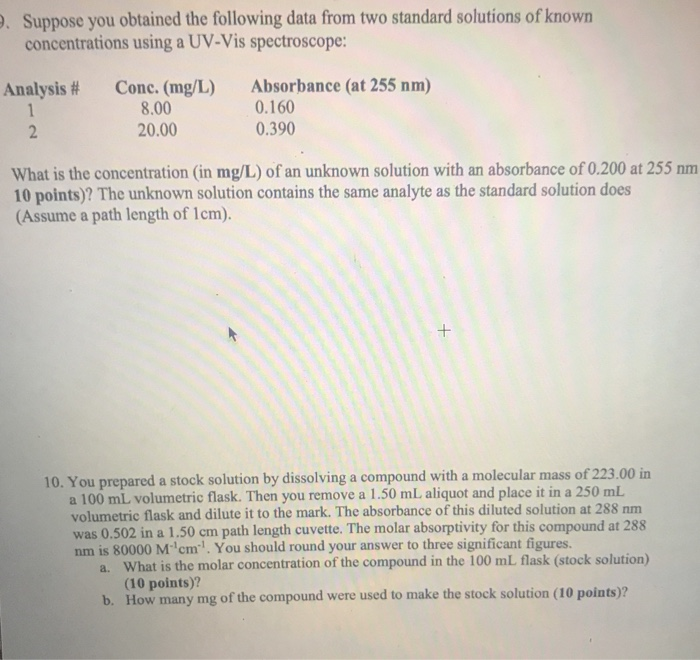Suppose you obtained the following data from two standard solutions of known concentrations using a UV-Vis spectroscope: Analysis # Conc. (mg/L) 8.00 20.00 Absorbance (at 255 nm) 0.160 0.390 What is the concentration (in mg/L) of an unknown solution with an absorbance of 0.200 at 255 nm 10 points)? The unknown solution contains the same analyte as the standard solution does (Assume a path length of 1cm). 10. You prepared a stock solution by dissolving a compound with a molecular mass of 223.00 in a 100 mL volumetric flask. Then you remove a 1.50 mL aliquot and place it in a 250 mL volumetric flask and dilute it to the mark. The absorbance of this diluted solution at 288 nm was 0.502 in a 1.50 cm path length cuvette. The molar absorptivity for this compound at 288 nm is 80000 M'cm'. You should round your answer to three significant figures a. What is the molar concentration of the compound in the 100 ml flask (stock solution) (10 points)? b. How many mg of the compound were used to make the stock solution (10 points)?

The concentration of unknown solutions is calculated below: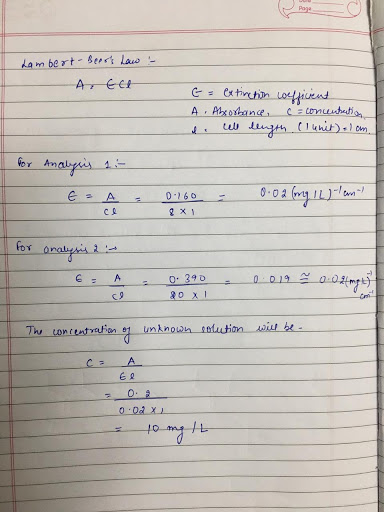#### Earn Coin

Coins can be redeemed for fabulous gifts.

Similar Homework Help Questions
• ### An unknown amount of a compound with a molecular mass of 294.46 g/mol is dissolved in...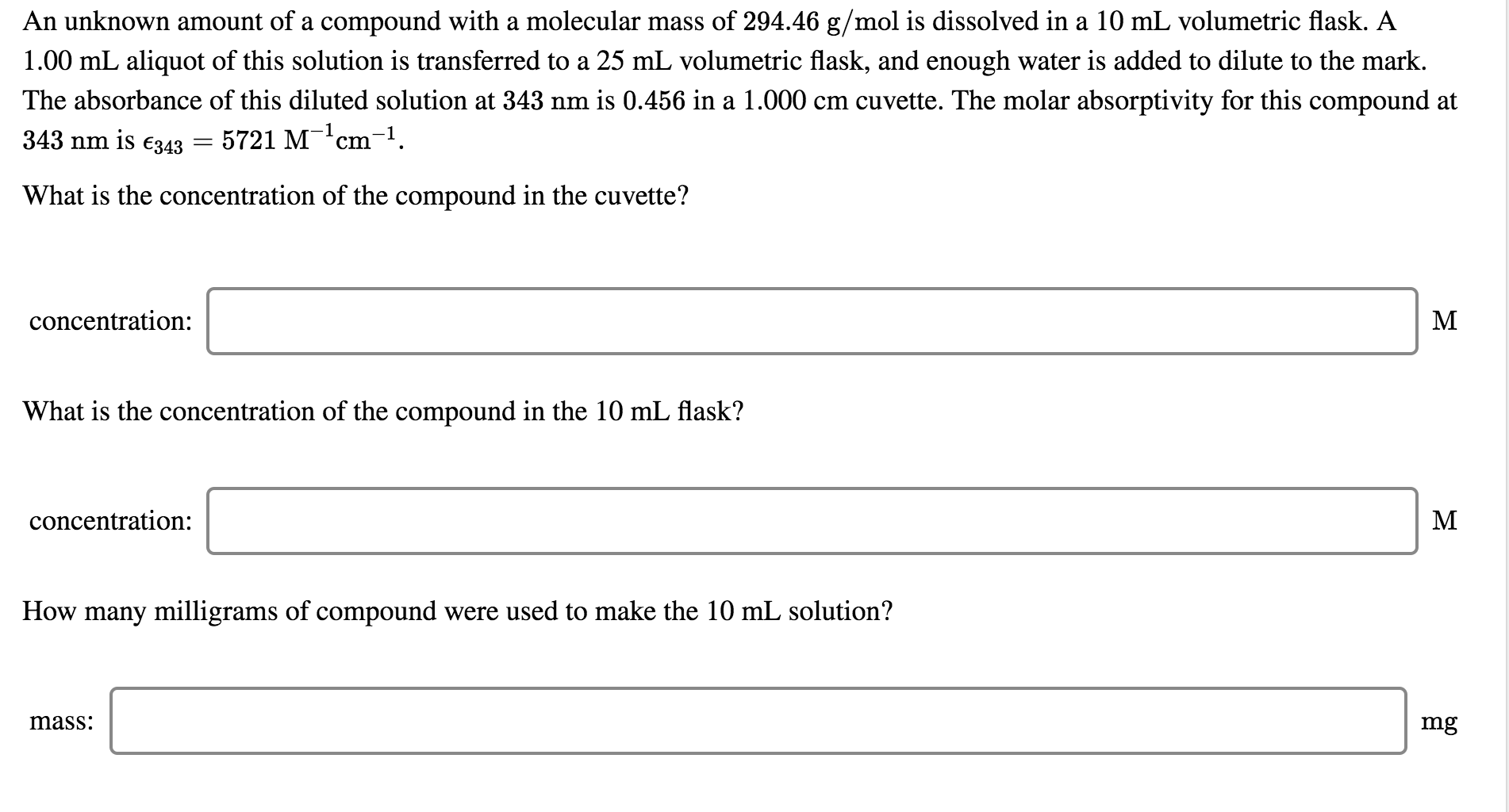An unknown amount of a compound with a molecular mass of 294.46 g/mol is dissolved in a 10 mL volumetric flask. A 1.00 mL aliquot of this solution is transferred to a 25 mL volumetric flask, and enough water is added to dilute to the mark. The absorbance of this diluted solution at 343 nm is 0.456 in a 1.000 cm cuvette. The molar absorptivity for this compound at 343 nm is €343 = 5721 M-?cm-1. What is the concentration...

• ### An unknown amount of a compound with a molecular mass of 270.93 g/mol is dissolved in...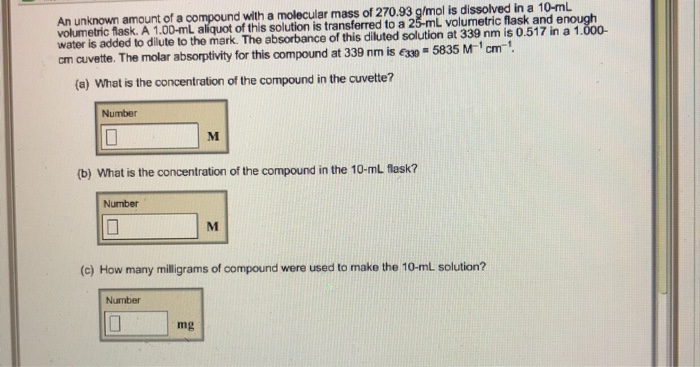An unknown amount of a compound with a molecular mass of 270.93 g/mol is dissolved in a 10-mL volumetric flask. A 1.00-mL aliquot of this solution is transferred to a 25-mL volumetric flask and e water is added to dilute to the mark. The absorbance of this diluted solution at 339 nm is 0.517 in a 1 cm cuvette. The molar absorptivity for this compound at 339 nm is esso- 5835 M-1cm-1 (a) What is the concentration of the compound...

• ### C. Problems 1Explain the difference between transmittance, absorbance, and molar absorptivity Which one is proporti...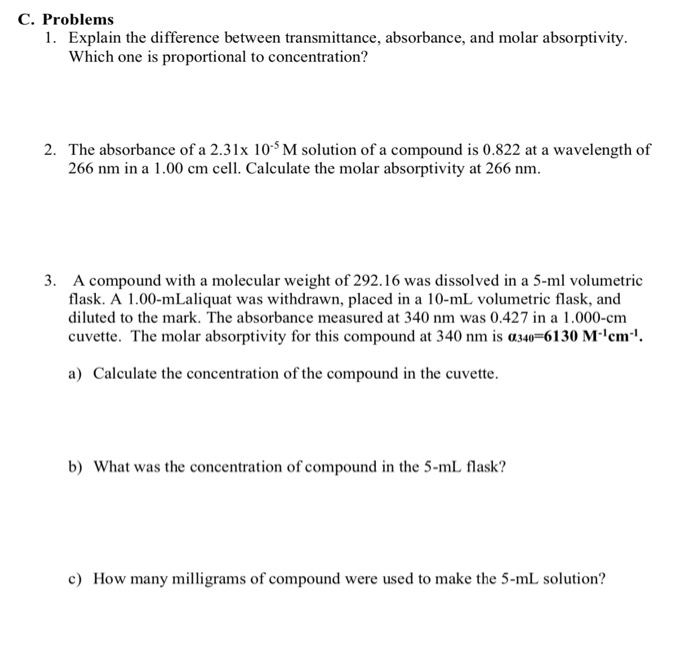C. Problems 1Explain the difference between transmittance, absorbance, and molar absorptivity Which one is proportional to concentration? 2. The absorbance of a 2.3lx 10 M solution of a compound is 0.822 at a wavelength of 266 nm in a 1.00 cm cell. Calculate the molar absorptivity at 266 nm 3. A compound with a molecular weight of 292.16 was dissolved in a 5-ml volumetric flask. A 1.00-mLaliquat was withdrawn, placed in a 10-mL volumetric flask, and diluted to the mark....

• ### An unknown amount of a compound with a molecular mass of 259.45 g/mol is dissolved in...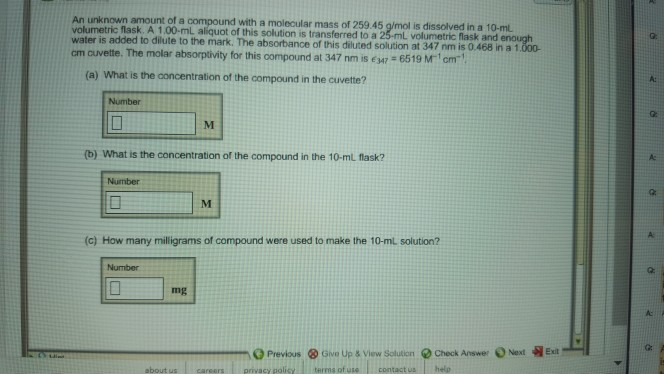An unknown amount of a compound with a molecular mass of 259.45 g/mol is dissolved in a 10-mL volumetric flask. A 1,00-mL aliquot of this solution is transferred to a 2 L volumetric flask and o the mark. The absorbance of this diluted solution at 347 nm is 0.468 in a 1 cm cuvette. The molar (a) What is the concentration of the compound in the cuvette? Number b) What is the concentration of the compound in the 10-ml flask?...

• ### Question 8 Status: Tries remaining: 21 Points possible: 1.00 Suppose you measure the absorbance of a...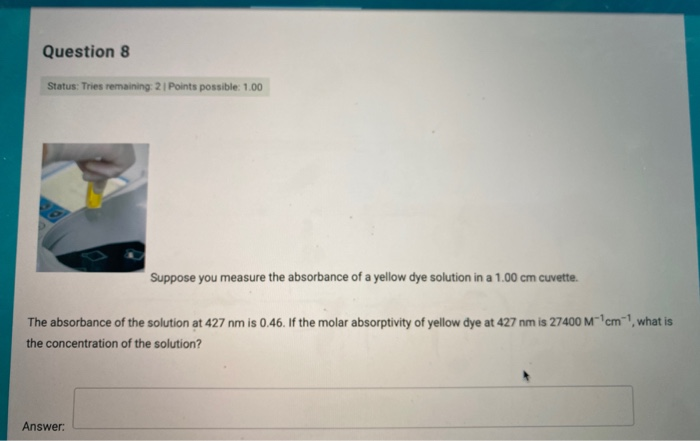Question 8 Status: Tries remaining: 21 Points possible: 1.00 Suppose you measure the absorbance of a yellow dye solution in a 1.00 cm cuvette. cm what is The absorbance of the solution at 427 nm is 0.46. If the molar absorptivity of yellow dye at 427 nm is 27400 M the concentration of the solution? Answer: Question 9 Status: Tries remaining: 2 Points possible: 1.00 If you plot the absorbance measurements of solutions vs. their concentrations, what is the slope...

• ### You prepared standard benzoic acid and caffeine solutions that allowed you to produce four standard curves...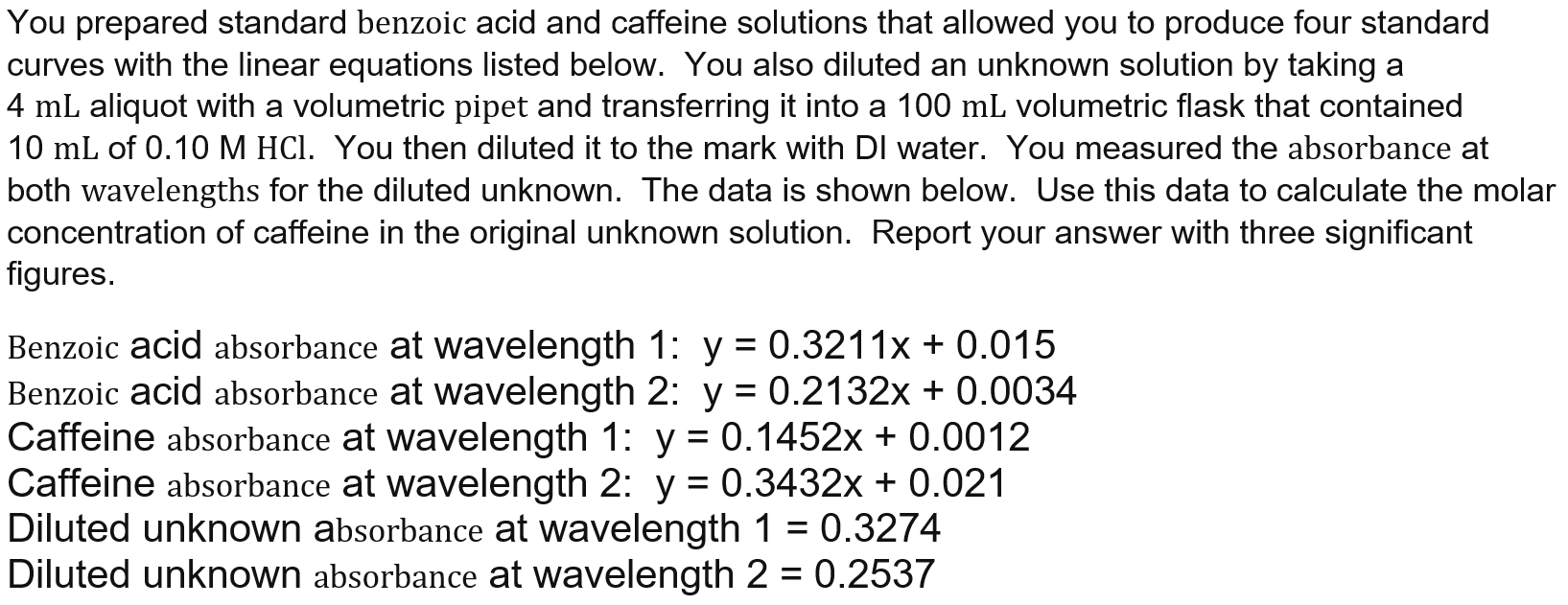You prepared standard benzoic acid and caffeine solutions that allowed you to produce four standard curves with the linear equations listed below. You also diluted an unknown solution by taking a 4 mL aliquot with a volumetric pipet and transferring it into a 100 mL volumetric flask that contained 10 mL of 0.10 M HCl. You then diluted it to the mark with Dl water. You measured the absorbance at both wavelengths for the diluted unknown. The data is shown...

• ### An unknown amount of a compound with a molecular mass of 274.11 g/mol 274.11 g/mol is...

An unknown amount of a compound with a molecular mass of 274.11 g/mol 274.11 g/mol is dissolved in a 10 mL volumetric flask. A 1.00 mL aliquot of this solution is transferred to a 25 mL volumetric flask, and enough water is added to dilute to the mark. The absorbance of this diluted solution at 325 nm 325 nm is 0.523 0.523 in a 1.000 cm cuvet. The molar absorptivity for this compound at 325 nm 325 nm is ?...

• ### Name: Determination of Solution Concentration by Spectrophotometry PRELAB QUESTIONS 1. A student measures the absorbance of...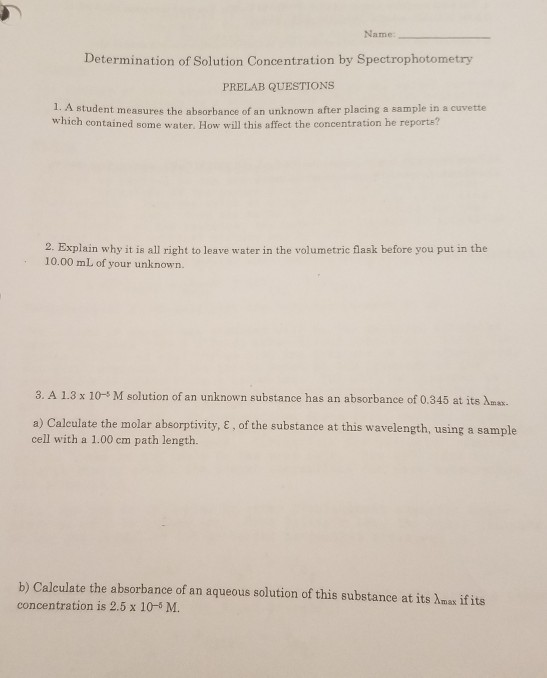Name: Determination of Solution Concentration by Spectrophotometry PRELAB QUESTIONS 1. A student measures the absorbance of an unknown after placing a sample in a cuvette which contained some water. How will this affect the concentration he reports? 2. Explain why it is all right to leave water in the volumetric flask before you put in the 10.00 mL of your unknown. 3. A 1.3 x 10-M solution of an unknown substance has an absorbance of 0.345 at its max. a)...

• ### 1. A series of solutions, containing cobalt (I1) chloride were prepared with concentrations shown below. Using...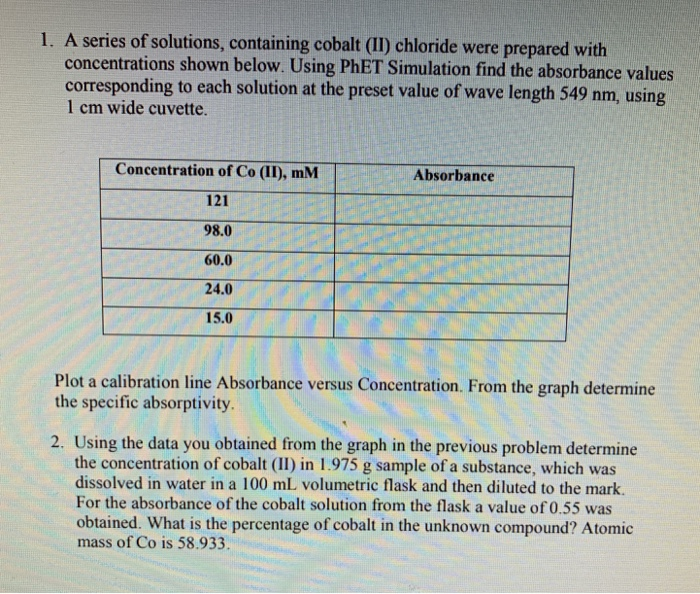1. A series of solutions, containing cobalt (I1) chloride were prepared with concentrations shown below. Using PhET Simulation find the absorbance values corresponding to each solution at the preset value of wave length 549 nm, using 1 cm wide cuvette. Concentration of Co (II), mM 121 98.0 60.0 24.0 15.0 Absorbance Plot a calibration line Absorbance versus Concentration. From the graph determine the specific absorptivity. 2. Using the data you obtained from the graph in the previous problem determine the...

• ### Please type in the Computer so I can be able to read it. A student need...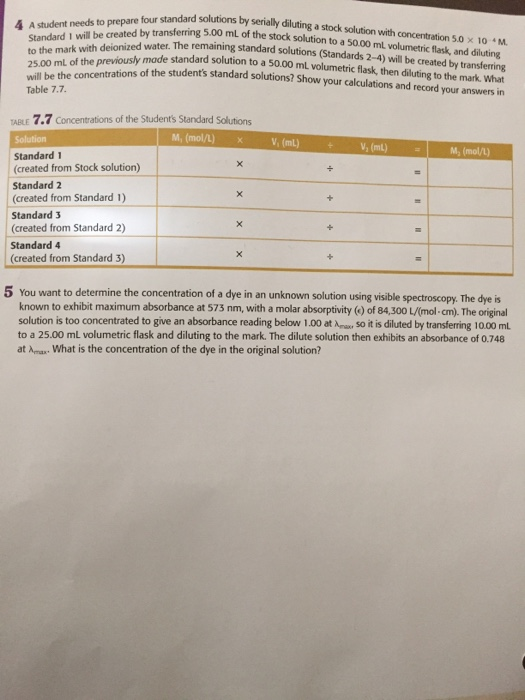Please type in the Computer so I can be able to read it. A student need to prepare four standard solutions by serially diluting a stock solution with concentration 5.0 times 10^-4 N. Standard I will be created by transferring 5.00 mL of the stock solution to the mark with deionized water. The remaining standard solutions (Standards 2 - 4) will be created by transferring 25.00 mL of the previously made standard solution to a 50.00 mL volumetric flask, then...